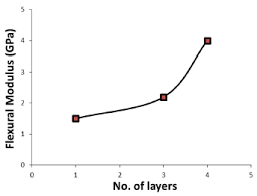## How to Calculate and Solve for Flexural Strength for Rectangular Cross-section in Defects | CeramicsThe image above represents flexural strength for rectangular cross-section in defects.

To compute for flexural strength for rectangular cross-section in defect, four essential parameters are needed and these parameters are Load at fracture (Ff), Length of the cross-section (b), Width of the cross-section (d) and Distance between support points (L).

The formula for calculating flexural strength for rectangular cross-section in defects:

σfs = 3FfL / 2bd²

Where:

σfs = Flexural Strength
L = Distance between Support Points
b = Length of Cross-section
d = Width of Cross-section

Let’s solve an example;
Find the flexural strength when the load at fracture is 10, the length of cross-section is 7, width of cross-section is 9 and the distance between support points is 21.

This implies that;

Ff = Load at Fracture = 10
L = Distance between Support Points = 21
b = Length of Cross-section = 7
d = Width of Cross-section = 9

σfs = 3FfL / 2bd²
σfr = 3(10)(21) / 2(7)(9)²
σfr = (630) / 2(7)(81)
σfr = (630) / (1134)
σfr = 0.55

Therefore, the flexural strength for rectangular cross-section in defect is 0.55 Pa.

Calculating the Distance Between Support Points when the Flexural Strength for Rectangular Cross-Section in Defects, Load at Fracture, length of the cross-section and width of cross-section is Given.

L = σfs x 2bd2 / 3Ff

Where;

L = Distance between Support Points
σfs = Flexural Strength
b = Length of Cross-section
d = Width of Cross-section

Let’s solve an example;
Find the distance between support points when the flexural strength is 20, the load at fracture is 12, the length of cross-section is 7 and the width of cross-section is 9.

This implies that;

σfs = Flexural Strength = 20
Ff = Load at Fracture = 12
b = Length of Cross-section = 7
d = Width of Cross-section = 9

L = σfs x 2bd2 / 3Ff
L = 20 x 2 x 7 x 92 / 3 x 12
L = 280 x 81 / 36
L = 22680 / 36
L = 630

Therefore, the distance between support points is 630.